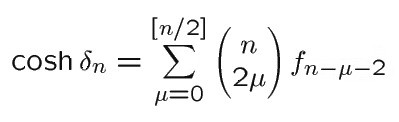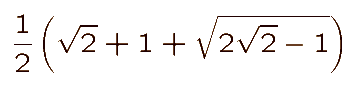# Coxeter on FIRMAMENT

It is known [H.S.M. Coxeter, 'Loxodromic Sequences of Tangent Spheres', Æquationes Mathematicæ, 1 (1968), pp. 112-117] that, for a sequence of circles sn such that every 4 consecutive members are mutually tangent, the inversive distance d n between s0 and sn (or between s m and s m+n for any m ) is given in terms of the Fibonacci numbers fn : 1, 1, 2, 3, 5, ..., by the formulaFor the analogous sequence of spheres, such that every 5 consecutive members are mutually tangent, a prize is offered to the first person who provides the analogous formula for the inversive distances between pairs of the spheres. Meanwhile, by taking one pair of adjacent 'spheres' to be a pair of parallel planes, one easily finds that the values of cosh dn are

 n = 1, 2, 3, 4, 5, 6, 7, cosh dn = 1, 1, 1, 1, 5, 7, 13.

John Robinson's sculpture FIRMAMENT is based on seven such spheres whose radii are in geometric progression; that is, the seven radii are proportional to

1/x3, 1/x2, 1/ x, 1, x, x2, x3 ,

where x is the root, between 1 and 2, of the quintic equation x5 - x4 - x3 - x2 - x + 1 = 0. This equation has a root -1 and the remaining quartic is easily solvable as a quadratic in x + 1/x to give x asor approximately 1.8832. This gives the radii previously described.

Donald Coxeter, January 1997

Back to Firmament

© Mathematics and Knots/Edition Limitee 1996 - 2002
This material may be used freely for educational, artistic and scientific purposes, but may not be used for commercial purposes, for profit or in texts without the permission of the publishers.